## The battery is now disconnected from the plates and the separation of the plates is doubled ( = 0.78 cm). What is the energy stored in this

Question

The battery is now disconnected from the plates and the separation of the plates is doubled ( = 0.78 cm). What is the energy stored in this new capacitor?

in progress 0
3 months 2021-09-02T05:12:52+00:00 1 Answers 1 views 0

The energy stored in this new capacitor is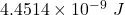Explanation:

Suppose, Two parallel plates, each having area A = 2180 cm² are connected to the terminals of a battery of voltage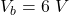as shown. The plates are separated by a distance d = 0.39 cm.

We need to calculate the charge

Using formula of capacitance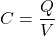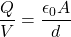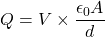Put the value into the formula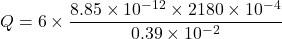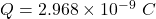The distance between the plates is doubled.

We need to calculate the new capacitance

Using formula of capacitance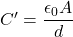Put the value into the formula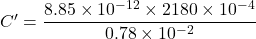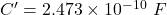We need to calculate the energy stored in this new capacitor

Using formula of energy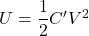Put the value into the formula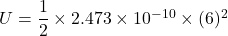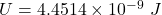Hence, The energy stored in this new capacitor is